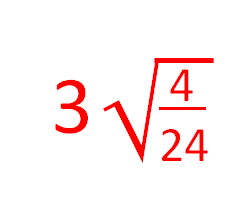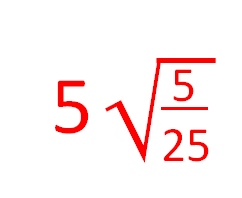# Rationalising Denominators (2)

In this worksheet, students rationalise the denominator of a fraction, giving answers as surds if necessary.Key stage:  KS 4

Curriculum topic:  Number

Curriculum subtopic:  Calculate Exactly with Fractions and Multiples of Pi

Difficulty level:### QUESTION 1 of 10

When we rationalise the denominator of a fraction that contains roots, we aim to remove the surd from the bottom of the fraction.

Remember that

√a x √a = √aa = √ =a

Example

Simplify by rationalising the denominator of this fraction giving your answer as a surd in the form a√b, where a and b are integers and b is as small as possible, or if the answer is a whole number, just state the whole number.4 24

simplifies to

 1 6

So the question is really asking us to rationalise

 3 √6

Multiply top and bottom by √6 to get

 3√6 √6√6

This simplifies to

 3√6 √36

which simplifies to

 3√6 6

which simplifies to

 √6 2

or ½√6

Simplify by rationalising the denominator of this fraction giving your answer as a surd in the form a√b, where a and b are integers and b is as small as possible, or if the answer is a whole number, just state the whole number.(√2)/16

2√8

2

Simplify by rationalising the denominator of this fraction giving your answer as a surd in the form a√b, where a and b are integers and b is as small as possible, or if the answer is a whole number, just state the whole number.(√5)/7

2√7

√7

Simplify by rationalising the denominator of this fraction giving your answer as a surd in the form a√b, where a and b are integers and b is as small as possible, or if the answer is a whole number, just state the whole number.(√6)/3

2√3

√6

Simplify by rationalising the denominator of this fraction giving your answer as a surd in the form a√b, where a and b are integers and b is as small as possible, or if the answer is a whole number, just state the whole number.(√5)/5

5√5

√5

Simplify by rationalising the denominator of this fraction giving your answer as a surd in the form a√b, where a and b are integers and b is as small as possible, or if the answer is a whole number, just state the whole number.(√4)/5

2

√2

Simplify by rationalising the denominator of this fraction giving your answer as a surd in the form a√b, where a and b are integers and b is as small as possible, or if the answer is a whole number, just state the whole number.4

4√4

√4

Simplify by rationalising the denominator of this fraction giving your answer as a surd in the form a√b, where a and b are integers and b is as small as possible, or if the answer is a whole number, just state the whole number.(√7)/2

4/7√14

√7

Simplify by rationalising the denominator of this fraction giving your answer as a surd in the form a√b, where a and b are integers and b is as small as possible, or if the answer is a whole number, just state the whole number.4(√5)/5

4√5

√5

Simplify by rationalising the denominator of this fraction giving your answer as a surd in the form a√b, where a and b are integers and b is as small as possible, or if the answer is a whole number, just state the whole number.2(√3)/3

4√12

(√12)/3

Simplify by rationalising the denominator of this fraction giving your answer as a surd in the form a√b, where a and b are integers and b is as small as possible, or if the answer is a whole number, just state the whole number.(√20)/4

½√5

(√5)/4

• Question 1

Simplify by rationalising the denominator of this fraction giving your answer as a surd in the form a√b, where a and b are integers and b is as small as possible, or if the answer is a whole number, just state the whole number.2√8
EDDIE SAYS
3/24 reduces to 1/8
Multiply top and bottom by √8
• Question 2

Simplify by rationalising the denominator of this fraction giving your answer as a surd in the form a√b, where a and b are integers and b is as small as possible, or if the answer is a whole number, just state the whole number.√7
EDDIE SAYS
5/35 reduces to 1/7
Multiply top and bottom by √7
• Question 3

Simplify by rationalising the denominator of this fraction giving your answer as a surd in the form a√b, where a and b are integers and b is as small as possible, or if the answer is a whole number, just state the whole number.(√6)/3
EDDIE SAYS
5/30 reduces to 1/6
Multiply top and bottom by √6
• Question 4

Simplify by rationalising the denominator of this fraction giving your answer as a surd in the form a√b, where a and b are integers and b is as small as possible, or if the answer is a whole number, just state the whole number.√5
EDDIE SAYS
5/25 reduces to 1/5
Multiply top and bottom by √5
• Question 5

Simplify by rationalising the denominator of this fraction giving your answer as a surd in the form a√b, where a and b are integers and b is as small as possible, or if the answer is a whole number, just state the whole number.2
EDDIE SAYS
The square root of 4/25 is 2/5
• Question 6

Simplify by rationalising the denominator of this fraction giving your answer as a surd in the form a√b, where a and b are integers and b is as small as possible, or if the answer is a whole number, just state the whole number.4
EDDIE SAYS
4/4 reduces to 1
√1 = 1
• Question 7

Simplify by rationalising the denominator of this fraction giving your answer as a surd in the form a√b, where a and b are integers and b is as small as possible, or if the answer is a whole number, just state the whole number.4/7√14
EDDIE SAYS
4/14 reduces to 2/7
Multiply top and bottom by √7
• Question 8

Simplify by rationalising the denominator of this fraction giving your answer as a surd in the form a√b, where a and b are integers and b is as small as possible, or if the answer is a whole number, just state the whole number.4(√5)/5
EDDIE SAYS
3/15 reduces to 1/5
Multiply top and bottom by √5
• Question 9

Simplify by rationalising the denominator of this fraction giving your answer as a surd in the form a√b, where a and b are integers and b is as small as possible, or if the answer is a whole number, just state the whole number.2(√3)/3
EDDIE SAYS
3/36 reduces to 1/12
Multiply top and bottom by √12 Remember 12 = 4 × 3
• Question 10

Simplify by rationalising the denominator of this fraction giving your answer as a surd in the form a√b, where a and b are integers and b is as small as possible, or if the answer is a whole number, just state the whole number.½√5
EDDIE SAYS
4/80 reduces to 1/20
Multiply top and bottom by √20 Remember 20 = 4 × 5
---- OR ----

Sign up for a £1 trial so you can track and measure your child's progress on this activity.

### What is EdPlace?

We're your National Curriculum aligned online education content provider helping each child succeed in English, maths and science from year 1 to GCSE. With an EdPlace account you’ll be able to track and measure progress, helping each child achieve their best. We build confidence and attainment by personalising each child’s learning at a level that suits them.